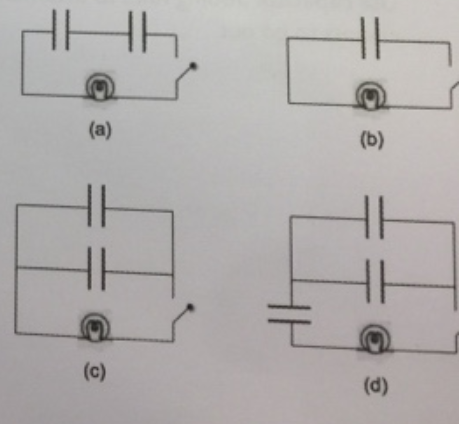# Problem: The capacitors in each circuit (a) through (d) pictured to the right, discharge when the switch closes at t = 0 seconds. Rank in order from largest to smallest, the length of time it takes for the circuit to discharge (i.e., the time constant for each circuit). The individual capacitors all have the same capacitance, and the light bulbs are identical in all circuits.

###### FREE Expert Solution

To rank, consider the time constant to be RC

The time constant is a function of capacitance.

(a) Time constant = RC/2

100% (142 ratings)###### Problem Details

The capacitors in each circuit (a) through (d) pictured to the right, discharge when the switch closes at t = 0 seconds. Rank in order from largest to smallest, the length of time it takes for the circuit to discharge (i.e., the time constant for each circuit). The individual capacitors all have the same capacitance, and the light bulbs are identical in all circuits.Frequently Asked Questions

What scientific concept do you need to know in order to solve this problem?

Our tutors have indicated that to solve this problem you will need to apply the Capacitors & Capacitance concept. You can view video lessons to learn Capacitors & Capacitance. Or if you need more Capacitors & Capacitance practice, you can also practice Capacitors & Capacitance practice problems.

What professor is this problem relevant for?

Based on our data, we think this problem is relevant for Professor Stallings' class at UCSD.